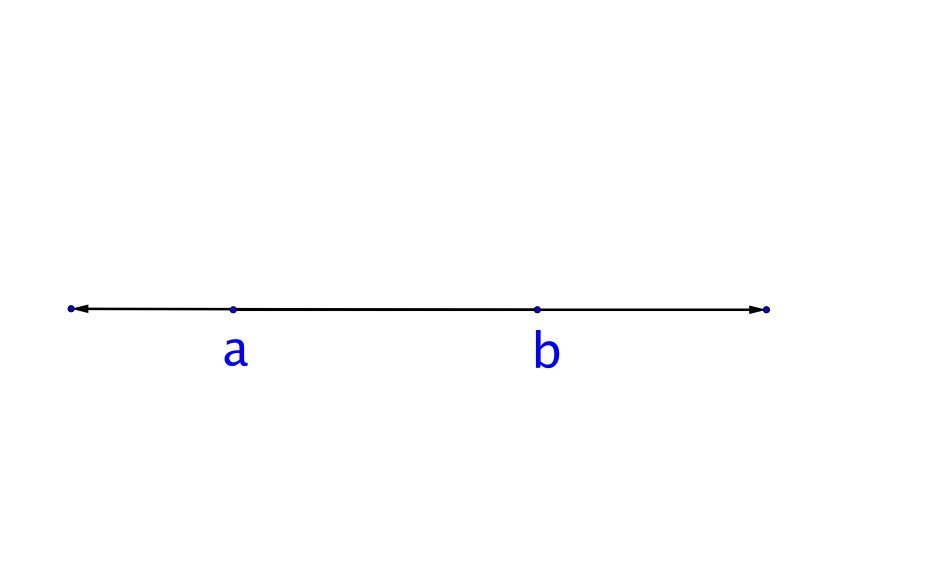# LINR 2 | Lesson 2 | Explore (Values on a Number-Line)# Explore Values on a Number Line

Given any two numbers on a number line, $$a$$ and $$b,$$ where $$a$$ is to the left of $$b$$ as shown below.  What mathematical statements can you make to describe numbers to the left of $$a,$$ numbers between $$a$$ and $$b,$$ and numbers to the right of $$b$$ ?Explore using Desmos inequalities.  Go to Desmos  https://student.desmos.com/join/pcbzp4 and select “Continue without signing in”.